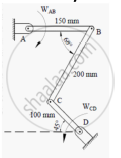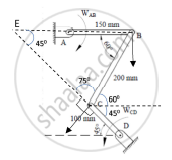Advertisement Remove all ads

# The Link Cd of the Mechanism Shown in Figure 7 is Rotating in Counterclockwise Direction at an Angular Velocity of 5 Rad/S. for the Given Instance, Determine the Angular Velocity of Link Ab. - Engineering Mechanics

Answer in Brief

The link CD of the mechanism shown in Figure 7 is rotating in counterclockwise direction at an angular velocity of 5 rad/s. For the given instance, determine the angular velocity of link AB.Advertisement Remove all ads

#### SolutionIn ΔEBC,
Using Sine rule,

(EB)/(sin(75)) -  (BC)/(sin(45) )-   (CE)/(sin(60)

EB = (200 xx sin (75))/(sin(45)) = 0.27 m               CE = (200 xx sin (60))/sin(45) = 0.24m

ωCD = VC x CD
V_C = 5/0.1= 50 m/s
ω_(BC) = V_C xx  CE = 50 x 0.24 = 12 rad/s
ω_(BC) = V_B xx EB

V_B = 12/(0.27) = 44.44m/s

ω_AB = V_B xx AB = 44.44 xx 0.15 = 6.666 rad/s

The angular velocity of link AB is 6.666 rad/s.

Concept: Instantaneous Center of Rotation for the Velocity
Is there an error in this question or solution?
Advertisement Remove all ads

#### APPEARS IN

Advertisement Remove all ads
Advertisement Remove all ads
Share
Notifications

View all notifications

Forgot password?